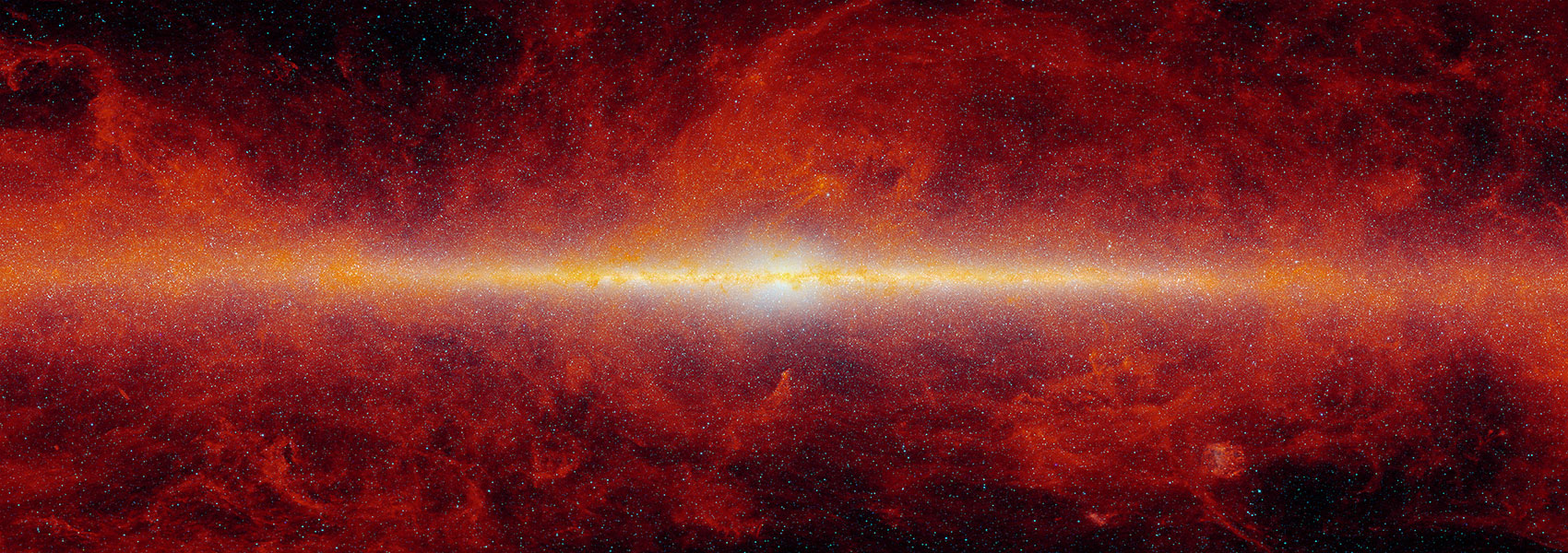# An 8.8 Minute Orbital Period Eclipsing Detached Double White Dwarf Binary

December 2020 • 2020ApJ...905L...7B

Authors • Burdge, Kevin B. • Coughlin, Michael W. • Fuller, Jim • Kaplan, David L. • Kulkarni, S. R. • Marsh, Thomas R. • Bellm, Eric C. • Dekany, Richard G. • Duev, Dmitry A. • Graham, Matthew J. • Mahabal, Ashish A. • Masci, Frank J. • Laher, Russ R. • Riddle, Reed • Soumagnac, Maayane T. • Prince, Thomas A.

Abstract • We report the discovery of ZTF J2243+5242, an eclipsing double white dwarf binary with an orbital period of just 8.8 minutes, the second known eclipsing binary with an orbital period of less than 10 minutes. The system likely consists of two low-mass white dwarfs and will merge in approximately 400,000 yr to form either an isolated hot subdwarf or an R Coronae Borealis star. Like its 6.91 minute counterpart, ZTF J1539+5027, ZTF J2243+5242 will be among the strongest gravitational-wave sources detectable by the space-based gravitational-wave detector the Laser Space Interferometer Antenna (LISA) because its gravitational-wave frequency falls near the peak of LISA's sensitivity. Based on its estimated distance of $d={2425}_{-114}^{+108}\,\mathrm{pc}$ , LISA should detect the source within its first few months of operation and achieve a signal-to-noise ratio of 63 ± 7 after 4 yr. We find component masses of ${M}_{A}={0.323}_{-0.047}^{+0.065}$ and ${M}_{B}={0.335}_{-0.054}^{+0.052}\,{M}_{\odot }$ , radii of ${R}_{A}={0.0298}_{-0.0012}^{+0.0013}$ and ${R}_{B}={0.0275}_{-0.0013}^{+0.0012}\,{R}_{\odot }$ , and effective temperatures of ${T}_{A}={26,300}_{-900}^{+1700}$ and ${T}_{B}={19,200}_{-900}^{+1500}\,{\rm{K}}$ . We determine all of these properties and the distance to this system using only photometric measurements, demonstrating a feasible way to estimate parameters for the large population of optically faint (r > 21 mAB) gravitational-wave sources that the Vera Rubin Observatory and LISA should identify.